# Find all second order partial derivatives of f(x, y) = (x² +3y)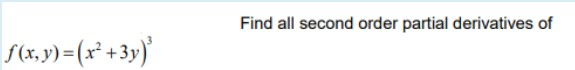Find all second order partial derivatives of f(x, y) = (x² +3y)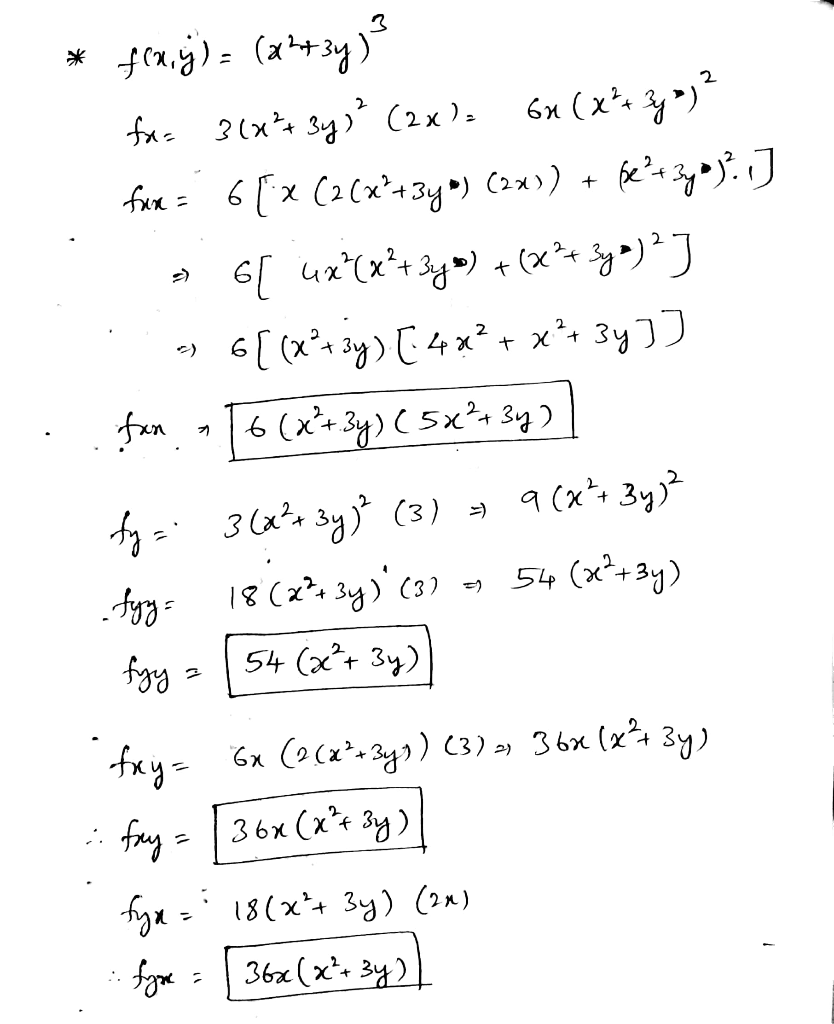##### Add Answer to: Find all second order partial derivatives of f(x, y) = (x² +3y)
Similar Homework Help Questions
• ### Find The indicated second-order Partial derivative. fxx(x,y) if f(x,y)=5x-3y+3 Find the indicated second-order partial derivative. fxx...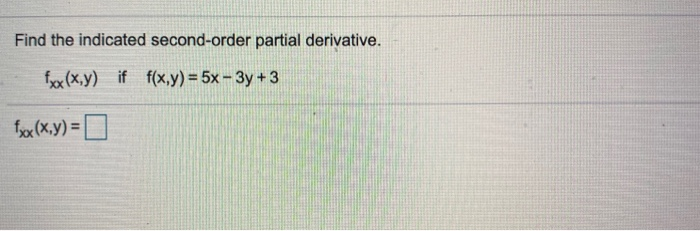Find The indicated second-order Partial derivative. fxx(x,y) if f(x,y)=5x-3y+3 Find the indicated second-order partial derivative. fxx (x,y) if f(x,y) = 5x - 3y + 3 fxx(x,y) =

• ### Find The indicated second-order Partial derivative. fxx(x,y) if f(x,y)=5x-3y+3 Find the indicated second-order partial derivative. fxx...Find The indicated second-order Partial derivative. fxx(x,y) if f(x,y)=5x-3y+3 Find the indicated second-order partial derivative. fxx (x,y) if f(x,y) = 5x - 3y + 3 fxx(x,y) =

• ### Find the first-order partial derivatives (fr. f,) and second-order partial derivatives (fxxıfyy, fxy, fyx) of the...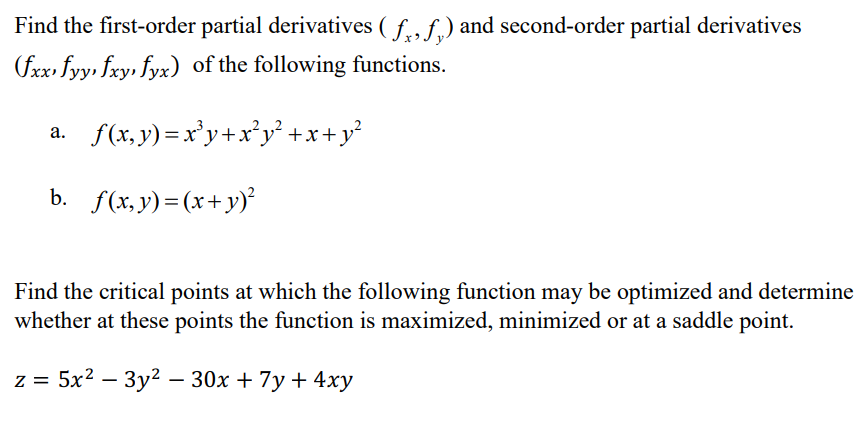Find the first-order partial derivatives (fr. f,) and second-order partial derivatives (fxxıfyy, fxy, fyx) of the following functions. a. f(x,y)=x’y+x’y? +x+y? b. f(x, y) = (x + y)? Find the critical points at which the following function may be optimized and determine whether at these points the function is maximized, minimized or at a saddle point. z = 5x2 – 3y2 – 30x + 7y + 4xy

• ### Find the four second-order partial derivatives for f(x, y) = 2x4,5 + 7x®y?. tyy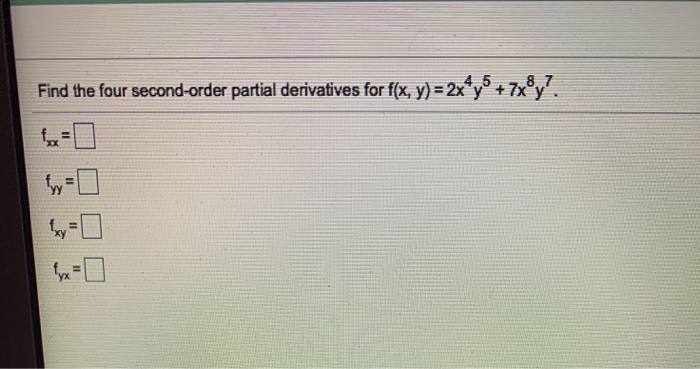Find the four second-order partial derivatives for f(x, y) = 2x4,5 + 7x®y?. tyy

• ### find all first partial derivatives f(x,y)= 5x^3+4y-3 Find all first partial derivatives. f(x, y) = 5x3...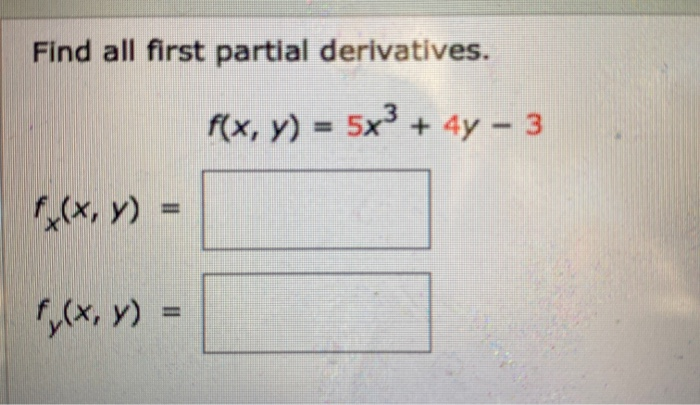find all first partial derivatives f(x,y)= 5x^3+4y-3 Find all first partial derivatives. f(x, y) = 5x3 + 4y - 3 f(x,y) = f(x,y) =

• ### the answers should look similar to the bottom picture please, thanks Find all the second-order partial...the answers should look similar to the bottom picture please, thanks Find all the second-order partial derivatives of the following function. 2x - y W= 2x2 + 2y 2w x² Find all the second-order partial derivatives of the following function. 3x - 3y W= 3x² + y Bw 54x3 - 162x?y - 54xy + 18y2 ox? (3x² + y) 3 w 18x? ay? (3x + y) = + 6x 3 54x3 + 27x? - 18xy - 3y (3x + y)...

• ### Problem 8. (1 point) (1 pt) Calculate all four second-order partial derivatives of f(x,y) = 2x²y...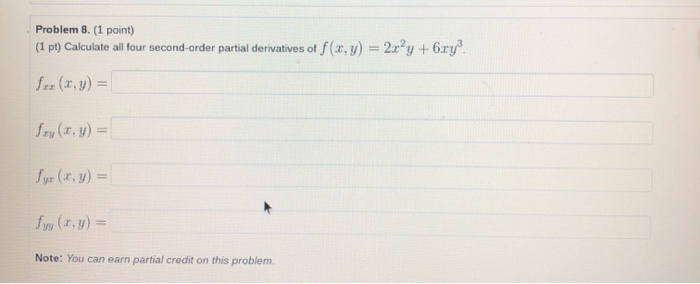Problem 8. (1 point) (1 pt) Calculate all four second-order partial derivatives of f(x,y) = 2x²y +6.ry? for (x,y) = Szy(x, y) = Syr (x, y) = fry (x. y) = Note: You can earn partial credit on this problem.

• ### Second Order Partial Derivatives

Confirm the mixed second order partial derivatives of f are the samef(x,y)= (x2+y2)/ (x2-y2)

• ### 4. (5 points) (a): Find all second partial derivatives of f(x, y) = re-2ry, (b): Find...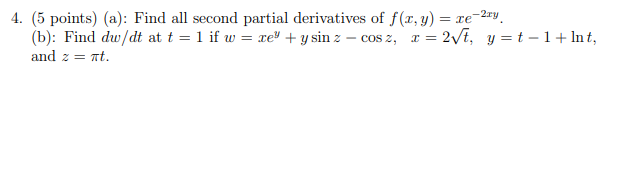4. (5 points) (a): Find all second partial derivatives of f(x, y) = re-2ry, (b): Find dw/dt at t = 1 if w = re + y sin z - cosz, r= 2/t, y=t-1+ lnt, and 2 = t.

• ### 4. Find the second partial derivatives for the function f(x,y) = x+yat (1,0). (6 Pts)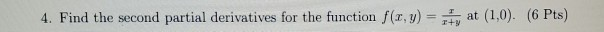4. Find the second partial derivatives for the function f(x,y) = x+yat (1,0). (6 Pts)

Free Homework App